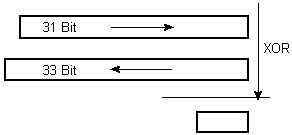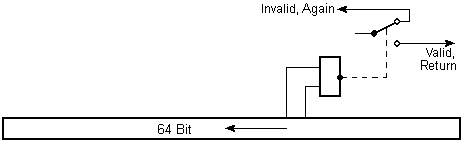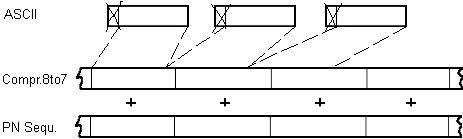November 24th, 2003
How it Works

### Feedback Shift Registers

Unlike the classic block codes e.g. DES or Rijndael which always scramble a block in several loops, here we have a stream cipher. The central element is a randomizer that generates a reproducible "noise" which is added to the plaintext byte by byte. This byte current has good statistical qualities. On average there are as many ones as zeros. The underlying mathematical polynomial division is implemented technically with multiple coupled feedback shift registers.

However, a single shift register is not sufficient, because its characteristic polynomial can be determined quite fast when only a couple of bits of the sequence have been recognized.Therefore two reversely circulating registers (31 bits and 33 bits) were selected as operands for a bitwise addition modulo 2 (XOR). A third register (64 bits) decides whether the value of the function is valid or gets rejected.#### 31-bit Register

This register has a sequence length of 2^31-1 = 2147483647. That is a prime number which guarantees that the following methods multiply this length and that short cycles caused by randomly caught factors won't occur.

The key selects a feedback polynomial from a list of primitive polynomials. All these polynomials are irreducible. Every register value is then part of a maximum ring yield.

#### 33-bit Register

The feedback polynomial for this register is derived from the key directly. This means that a maximum cycle arises here only by chance. The circulation sequences can be very much shorter but it is unknown when.

The advantage consists in the fact that the polynomial which determines the sequence is unknown and cannot be predicted either. 2147483648 different polynomials are possible in the current implementation.

#### 64-bit Register and Butt

This register operates exactly like the 33-bit register with a coincidental polynomial which is derived of the key directly. For performance reasons the number of the possible polynomials is confined also to 2147483648.

While the register circulates, a "butt" intrudes on the transmissions and detects the contents of two randomly selected bits. Only if these two bits are zero, the combination of 31 bit and 33 bit which was calculated above, is issued as return value of the function. Well, in the average 3/4 of all results are thrown away (sometimes only half of the results, but when this occurs is unknown again). The butt can select 528 various sub-sequences respectively.

### Key Generation with MD5

From the entered passphrase the MD5 algorithm calculates a 128-bit number, which are 16 bytes. Those initialize the start values for the circulating shift registers. After that the key is sent through the MD5 algorithm again to derive the feedback polynomials.

### ASCII Compression and Base64 Coding

The plaintext is pure a ASCII text. In order not to betray every eighth bit of the key sequence, the ASCII bytes are compressed to 7 bits before encoding and expanded after the deciphering again.The encoded byte stream still needs to be integrated in its carrier, namely into a html page. However, this won't work in the somewhat raw state, because none of the characters in the set of values between 128 and 255 are part of the ASCII code, and even in the standard ASCII section special signs could be re-coded, Dos2Unix changed or something else. Therefore the byte stream is cut into 6 bit pieces which are encoded with standard ASCII characters (Base64). It shouldn't be possible to disfigure the now resulting block on a html page by any code conversion.